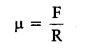# Define coefficient of friction and angle of friction and hence derive a relation between them

The coefficient of friction between any two surfaces in contact is defined as the ratio of the force of limiting friction and normal reaction between them.Angle with the resultant of force of limiting friction F and normal reaction R makes with the direction of normal reaction R is angle of reaction.